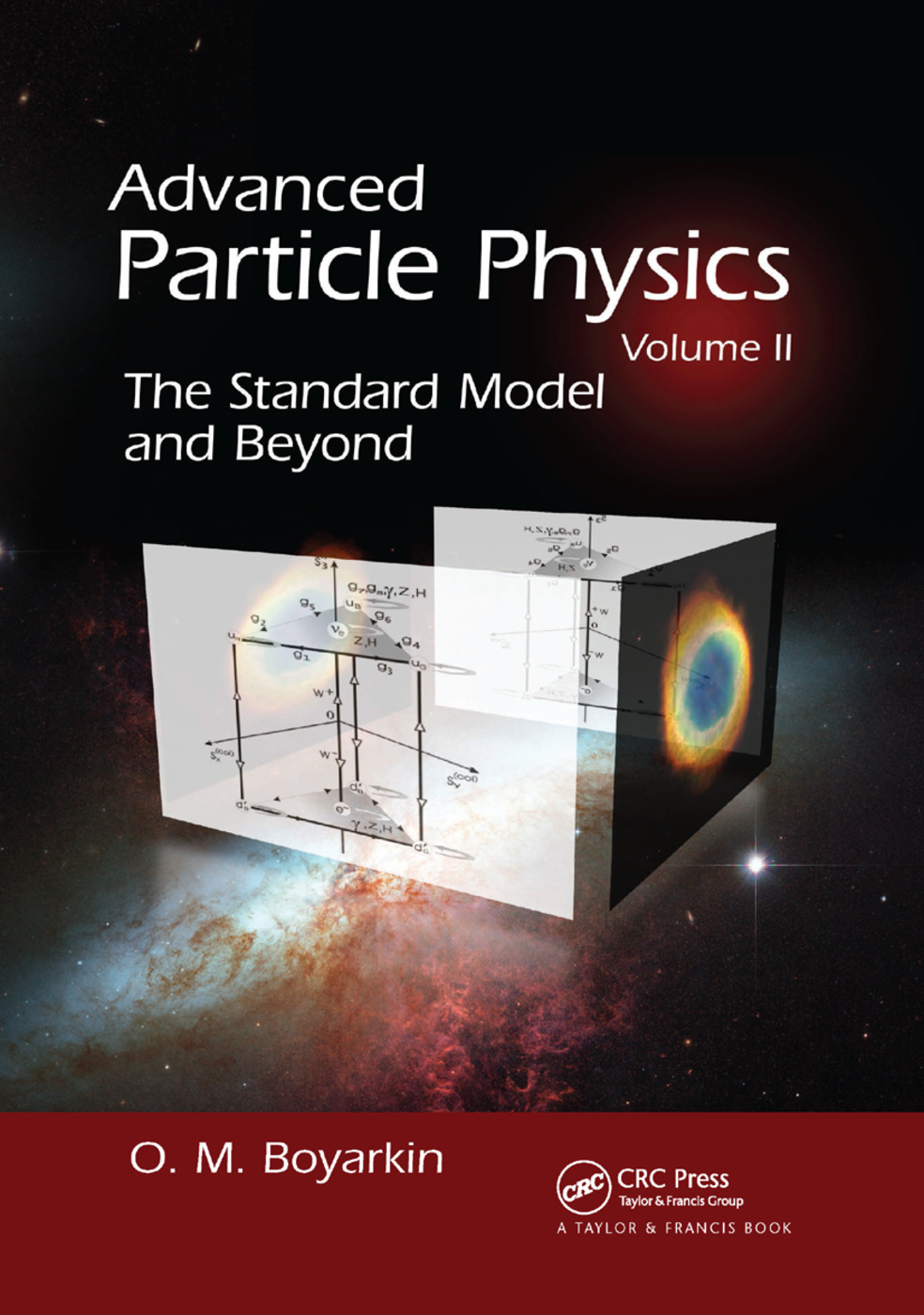The Standard Model and Beyond, 1st Edition

CRC Press

565 pages | 169 B/W Illus.

New in Paperback: 9781138374119
pub: 2018-09-10
\$71.00
x
Hardback: 9781439804162
pub: 2011-02-16
\$130.00
x
eBook (VitalSource) : 9780429191558
pub: 2011-02-16
from \$35.50

FREE Standard Shipping!

Description

Helping readers understand the complicated laws of nature, Advanced Particle Physics Volume II: The Standard Model and Beyond explains the calculations, experimental procedures, and measuring methods of particle physics, particularly quantum chromodynamics (QCD). It also discusses extensions to the Standard Model and the physics of massive neutrinos.

Divided into three parts, this volume begins with QCD. It explains the quantization scheme using functional integrals and investigates renormalization problems. The book also calculates cross sections of basic hard processes and covers nonperturbative methods, such as the lattice approach and QCD vacuum. The next part focuses on electroweak interactions, in which the author describes the Glashow–Weinberg–Salam theory and presents composite models and a left-right symmetric model as extensions to the Standard Model. The book concludes with chapters on massive neutrino physics that cover neutrino properties, neutrino oscillation in vacuum and matter, and solar and atmospheric neutrinos.

QUANTUM CHROMODYNAMICS

Canonical Quantization

Fundamental relations of chromodynamics

Gauge invariance in QCD

Quantization of free gluon field

Feynman rules in QCD with covariant gauge

Formalism of Functional Integration

Functional integral in quantum mechanics

Boson fields quantization by means of functional integrals method

Fermion fields quantization by means of functional integrals method

Functional formulation of QCD

Renormalization and Unitarity

Primitive divergent diagrams

Slavnov–Taylor identities

Ghosts and S-matrix unitarity

Renormalization of one-loop diagrams

Asymptotical Freedom

Callan–Symanzik equation

Evolution of effective coupling constant in QCD

Chiral Symmetries

Current algebras

Weak and electromagnetic currents of hadrons

Adler’s sum rules for neutrino reactions

Goldstone theorem

Hypothesis of partially conserved axial current

U(1)-problem

Anomalies

π0 → γγ decay

Chiral anomalies and chiral gauge theories

Anomalous breaking of scale invariance

Hard Processes in QCD

Deep inelastic scattering of leptons by proton

Parton distribution functions

Quark-gluon dynamics

Scaling violation. Altarelli–Parisi equation

Fragmentation functions

Drell–Yan process

Gauge bosons production at hadron collisions

Higgs bosons production

Lattice QCD

Lattice approach

Scalar field

Fermion fields

Geometric interpretation of gauge invariance

QCD action

Confinement

Quark-Gluon Plasma

Aggregate states of matter

Looking for QGP

QGP within holographic duality

Resume

QCD Vacuum

Kinks and breathers

Topology and vacuum in gauge theory

Vortices and monopoles

Instantons

Instanton physics

QCD Experimental Status

ELECTROWEAK INTERACTIONS

Glashow–Weinberg–Salam Theory

Choice of gauge group

Lagrangian in generalized renormalizable gauge

Four-fermion invariants and Fierz identities

Muon decays

(g − 2)μ anomaly

Effects of interference of weak and electromagnetic interactions

Neutrino-electron scattering

Neutrino-nucleon scattering

Physics beyond the Standard Model

Models, models

Multipole moments of gauge bosons

Left-right model

Left-right model in the making

NEUTRINO PHYSICS

Neutrino Properties

Neutrino mass in models with SU(2)L × U(1)Y gauge group

Multipole moments of neutrinos

Neutrino Oscillation in Vacuum

Description in formalism of de Broglie’s waves

Oscillations and uncertainty relation

Wave packet treatment of neutrino oscillations

Evolution equation for neutrino

Neutrino Oscillation in Matter

Neutrino motion in condensate matter

Neutrino oscillations in magnetic field

Neutrino oscillations in LRM

Solar Neutrinos

Sources of solar neutrinos

Detection of solar neutrinos

Analysis of neutrino observation data

Neutrino propagation through Earth

Atmospheric Neutrinos

Cosmic rays

Atmospheric neutrino production

Atmospheric neutrino detection

Results and Perspectives

References appear at the end of each section.